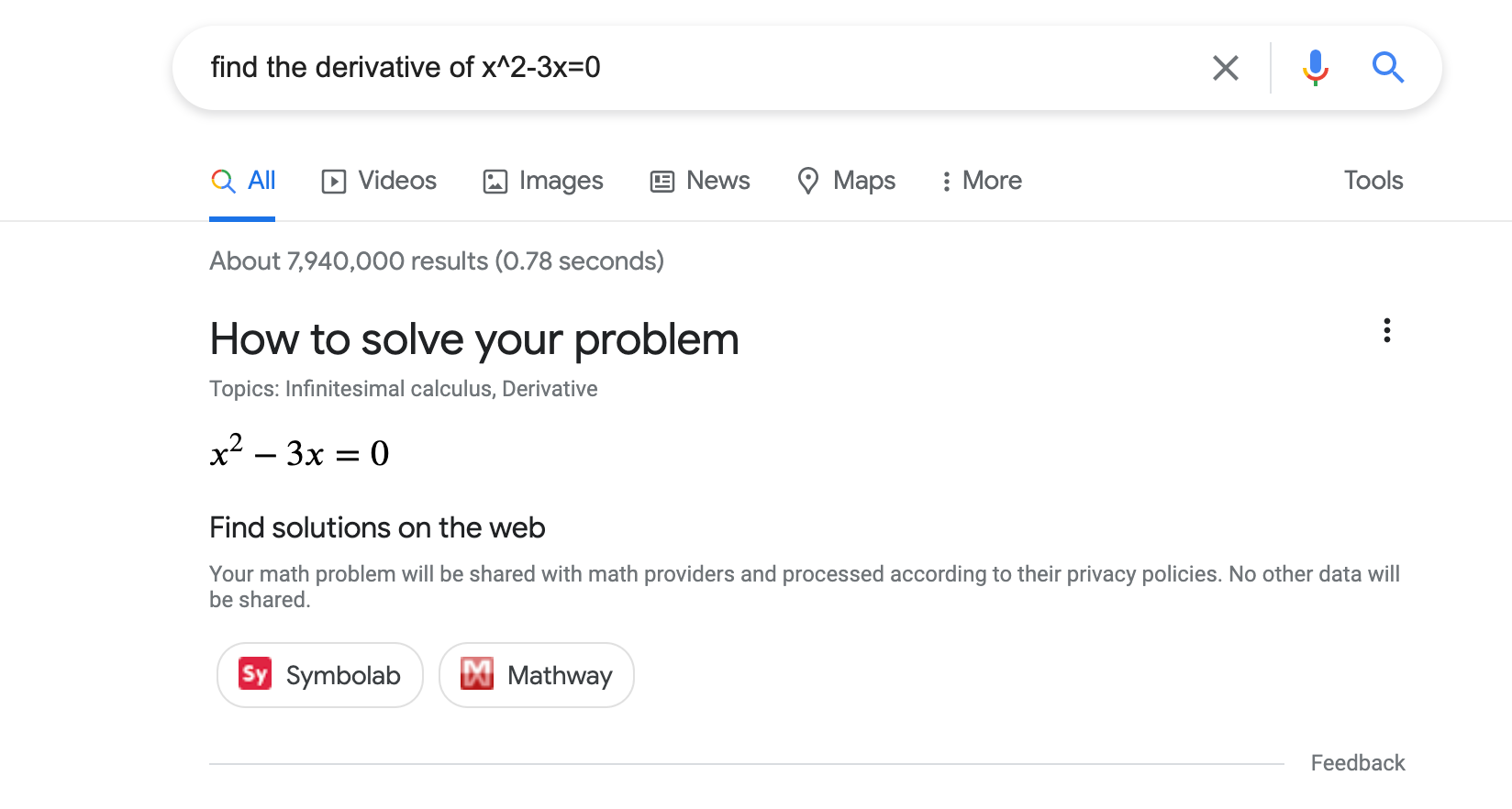# 數學解題工具 (MathSolver) 結構化資料## 如何新增結構化資料

1. 新增必要屬性。根據您使用的格式，瞭解要在網頁中的什麼位置插入結構化資料
2. 遵循指南規範
3. 使用複合式搜尋結果測試驗證程式碼，並修正所有重大錯誤。此外，我們也建議您修正工具中可能標記的任何非重大問題，因為這有助於改善結構化資料的品質 (但並非符合複合式搜尋結果的顯示條件)。
4. 部署幾個包含結構化資料的網頁，並使用網址檢查工具測試 Google 轉譯網頁的情形。請確認 Google 可以存取您的網頁，且網頁並未遭到 robots.txt 檔案或 noindex 標記封鎖，也未設有登入規定。如果網頁看起來沒問題，您可以要求 Google 重新檢索您的網址
5. 為了讓 Google 掌握日後的異動內容，建議您提交 Sitemap。您可以使用 Search Console Sitemap API 自動執行這項操作。

## 範例

### 一個解題工具動作

<html>
<title>An awesome math solver</title>
<body>
<script type="application/ld+json">
[
{
"@context": "https://schema.org",
"@type": ["MathSolver", "LearningResource"],
"name": "An awesome math solver",
"url": "https://www.mathdomain.com/",
"usageInfo": "https://www.mathdomain.com/privacy",
"inLanguage": "en",
"potentialAction": [{
"@type": "SolveMathAction",
"target": "https://mathdomain.com/solve?q={math_expression_string}",
"mathExpression-input": "required name=math_expression_string",
"eduQuestionType": ["Polynomial Equation","Derivative"]
}],
"learningResourceType": "Math solver"
},
{
"@context": "https://schema.org",
"@type": ["MathSolver", "LearningResource"],
"name": "Un solucionador de matemáticas increíble",
"url": "https://es.mathdomain.com/",
"usageInfo": "https://es.mathdomain.com/privacy",
"inLanguage": "es",
"potentialAction": [{
"@type": "SolveMathAction",
"target": "https://es.mathdomain.com/solve?q={math_expression_string}",
"mathExpression-input": "required name=math_expression_string",
"eduQuestionType": ["Polynomial Equation","Derivative"]
}],
"learningResourceType": "Math solver"
}
]
</script>
</body>
</html>

### 兩個解題工具動作

<html>
<title>An awesome math solver</title>
<body>
<script type="application/ld+json">
{
"@context": "https://schema.org",
"@type": ["MathSolver", "LearningResource"],
"name": "An awesome math solver",
"url": "https://www.mathdomain.com/",
"usageInfo": "https://www.mathdomain.com/privacy",
"inLanguage": "en",
"potentialAction": [{
"@type": "SolveMathAction",
"target": "https://mathdomain.com/solve?q={math_expression_string}",
"mathExpression-input": "required name=math_expression_string",
"eduQuestionType": "Polynomial Equation"
},
{
"@type": "SolveMathAction",
"target": "https://mathdomain.com/trig?q={math_expression_string}",
"mathExpression-input": "required name=math_expression_string",
"eduQuestionType": "Trigonometric Equation"
}],
"learningResourceType": "Math solver"
}
</script>
</body>
</html>

## 指南規範

### 技術指南

• 請將 MathSolver 結構化資料加在網站的首頁。
• 確認您的主機負載設定允許經常進行檢索
• 如果有多個不同網址都包含相同數學解題工具，請在每個網頁的標記中使用標準網址

### 內容指南

• 我們嚴禁偽裝成數學解題工具的宣傳內容，例如由聯盟計畫等第三方張貼的內容。
• 透過這項功能呈現數學解題工具內容時，您有責任確保內容正確無誤且品質優良。如果我們根據品質審查程序，發現您資料中的錯誤達到一定數量，可能會依照嚴重程度移除您的解題工具，直到問題解決。判斷錯誤的條件包括：
• 您的解題工具是否確實能夠解決該類型的問題。
• 解題工具所宣告的解法是否確實能解開該數學問題。

## 結構化資料類型定義

### MathSolver

MathSolver 這項工具能提供逐步解法說明，協助學生、教師和其他人解決數學問題。請將 MathSolver 結構化資料用於網站首頁。

schema.org/MathSolver 內提供 MathSolver 的完整定義。

potentialAction

SolveMathAction

{
"@type": "MathSolver",
"potentialAction": [{
"@type": "SolveMathAction",
"target": "https://mathdomain.com/solve?q={math_expression_string}",
"mathExpression-input": "required name=math_expression_string",
"eduQuestionType": "Polynomial Equation"
}]
}

potentialAction.mathExpression-input

Text

Google 傳送至您網站的數學運算式 (例如：x^2-3x=0) 的預留位置。接著您就可以「解答」數學運算式，其中可能涉及簡化、轉換或解析特定變數。這個字串可以採用多種格式，例如 LaTeX、Ascii-Math 或是任何可透過鍵盤撰寫的數學運算式。

Google 會以下列兩種形式之一傳送 math_expression_string

• (math_expression)'
• d/dvariable math_expression

Examples:

• (x^2+x)'
• d/dx (x^2+x)
• d/dy y^2+y

Integrals

Google will send a math_expression_string in one of two forms:

• \int math_expression
• \int_{from}^{to} math_expression

• \int x^2+x
• \int_{0}^{2} x^2+x

Google 會以下列兩種形式之一傳送 math_expression_string

• \lim math_expression
• \lim_{variable\rightarrowvalue} math_expression

• \lim_{x\rightarrow0} sin(x)/x
• \lim_{y\rightarrow\infty} sin(y)/y
• \lim sin(x)/x
url

URL

MathSolver 的網址。

usageInfo

URL

{
"@type": "MathSolver",
"usageInfo": "https://www.mathdomain.com/privacy"
}
potentialAction.target

EntryPoint

{
"@type": "MathSolver",
"potentialAction": [{
"@type": "SolveMathAction",
"target": "https://mathdomain.com/solve?q={math_expression_string}"
}]
}

inLanguage

Text

{
"@type": "MathSolver",
"inLanguage": "es"
}
assesses

HowTo 所解決的問題類型。如果您同時使用 MathSolver 標記和 HowTo 標記，那麼也請使用 assesses 屬性。

{
"@type": "MathSolver",
"assesses": "Polynomial Equation"
}
potentialAction.eduQuestionType

{
"@type": "SolveMathAction",
"eduQuestionType": "Polynomial Equation"
}


### LearningResource

LearningResource 表示標記主體為一項資源，可以用來協助學生、教師和其他教育學習人士。請將 LearningResource 用於網站首頁。

schema.org/LearningResource 內提供 LearningResource 的完整定義。

learningResourceType

Text

{
"@type": ["MathSolver", "LearningResource"],
"learningResourceType": "Math Solver"
}

## 問題類型定義

Absolute Value Equation

Algebra

Arc Length

Arithmetic

Biquadratic Equation

Calculus

Characteristic Polynomial

Circle

Derivative

Differential Equation

Distance

Eigenvalue

Eigenvector

Ellipse

Exponential Equation

Function

Function Composition

Geometry

Hyperbola

Inflection Point

Integral

Intercept

Limit

Line Equation

Linear Algebra

Linear Equation

Linear Inequality

Logarithmic Equation

Logarithmic Inequality

Matrix

{{1,2,5}, {3,-1,1}, {1,2,3}} 簡化列

Midpoint

Parabola

Parallel

Perpendicular

Polynomial Equation

Polynomial Expression

Polynomial Inequality

Quadratic Equation

Quadratic Expression

Quadratic Inequality

Radical Equation

Radical Inequality

Rational Equation

Rational Expression

Rational Inequality

Slope

Statistics

System of Equations

Trigonometry

[{ "type": "thumb-down", "id": "missingTheInformationINeed", "label":"缺少我需要的資訊" },{ "type": "thumb-down", "id": "tooComplicatedTooManySteps", "label":"過於複雜/步驟過多" },{ "type": "thumb-down", "id": "outOfDate", "label":"過時" },{ "type": "thumb-down", "id": "translationIssue", "label":"翻譯問題" },{ "type": "thumb-down", "id": "samplesCodeIssue", "label":"示例/程式碼問題" },{ "type": "thumb-down", "id": "otherDown", "label":"其他" }]
[{ "type": "thumb-up", "id": "easyToUnderstand", "label":"容易理解" },{ "type": "thumb-up", "id": "solvedMyProblem", "label":"確實解決了我的問題" },{ "type": "thumb-up", "id": "otherUp", "label":"其他" }]